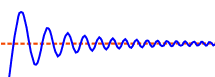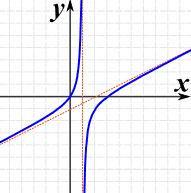# Asymptote

An asymptote is a line that a curve approaches, as it heads towards infinity:## Types

There are three types: horizontal, vertical and oblique:The direction can also be negative:The curve can approach from any side (such as from above or below for a horizontal asymptote),or may actually cross over (possibly many times), and even move away and back again.

The important point is that:

The distance between the curve and the asymptote tends to zero as they head to infinity (or −infinity)

## Horizontal AsymptotesIt is a Horizontal Asymptote when: as x goes to infinity (or −infinity) the curve approaches some constant value b

## Vertical AsymptotesIt is a Vertical Asymptote when: as x approaches some constant value c (from the left or right) then the curve goes towards infinity (or −infinity).

## Oblique AsymptotesIt is an Oblique Asymptote when: as x goes to infinity (or −infinity) then the curve goes towards a line y=mx+b (note: m is not zero as that is a Horizontal Asymptote).

### Example: (x2−3x)/(2x−2)The graph of (x2-3x)/(2x-2) has:

• A vertical asymptote at x=1
• An oblique asymptote: y=x/2 − 1

These questions will only make sense when you know Rational Expressions: